Tutorial on Graph Neural Networks for Computer Vision and Beyond (Part 1)

I’m answering questions that AI/ML/CV people not familiar with graphs or graph neural networks typically ask. I provide PyTorch examples to clarify the idea behind this relatively new and exciting kind of model.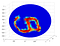A figure from (Bruna et al., ICLR, 2014) depicting an MNIST image on the 3D sphere. While it’s hard to adapt Convolutional Networks to classify spherical data, Graph Networks can naturally handle it. This is a toy example, but similar tasks arise in many real applications.

The questions addressed in this part of my tutorial are:

1. Why are graphs useful?
2. Why is it difficult to define convolution on graphs?
3. What makes a neural network a graph neural network?

To answer them, I’ll provide motivating examples, papers and Python code making it a tutorial on Graph Neural Networks (GNNs). Some basic knowledge of machine learning and computer vision is expected, however, I’ll provide some background and intuitive explanation as we go.

First of all, let’s briefly recall what is a graph? A graph G is a set of nodes (vertices) connected by directed/undirected edges. Nodes and edges typically come from some expert knowledge or intuition about the problem. So, it can be atoms in molecules, users in a social network, cities in a transportation system, players in team sport, neurons in the brain, interacting objects in a dynamic physical system, pixels, bounding boxes or segmentation masks in images. In other words, in many practical cases, it is actually you who gets to decide what are the nodes and edges in a graph.

In many practical cases, it is actually you who gets to decide what are the nodes and edges in a graph.

This is a very flexible data structure that generalizes many other data structures. For example, if there are no edges, then it becomes a set; if there are only “vertical” edges and any two nodes are connected by exactly one path, then we have a tree. Such flexibility is both good and bad as I’ll discuss in this tutorial.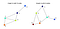Two undirected graphs with 5 and 6 nodes. The order of nodes is arbitrary.

1. Why graphs can be useful?

In the context of computer vision (CV) and machine learning (ML), studying graphs and the models to learn from them can give us at least four benefits:

1. We can become closer to solving important problems that previously were too challenging, such as: drug discovery for cancer (Veselkov et al., Nature, 2019); better understanding of the human brain connectome (Diez & Sepulcre, Nature Communications, 2019); materials discovery for energy and environmental challenges (Xie et al., Nature Communications, 2019).
2. In most CV/ML applications, data can be actually viewed as graphs even though you used to represent them as another data structure. Representing your data as graph(s) gives you a lot of flexibility and can give you a very different and interesting perspective on your problem. For instance, instead of learning from image pixels you can learn from “superpixels” as in (Liang et al., ECCV, 2016) and in our forthcoming BMVC paper. Graphs also let you impose a relational inductive bias in data — some prior knowledge you have about the problem. For instance, if you want to reason about a human pose, your relational bias can be a graph of skeleton joints of a human body (Yan et al., AAAI, 2018); or if you want to reason about videos, your relational bias can be a graph of moving bounding boxes (Wang & Gupta, ECCV, 2018). Another example can be representing facial landmarks as a graph (Antonakos et al., CVPR, 2015) to make reasoning about facial attributes and identity.
3. Your favourite neural network itself can be viewed as a graph, where nodes are neurons and edges are weights, or where nodes are layers and edges denote flow of forward/backward pass (in which case we are talking about a computational graph used in TensorFlow, PyTorch and other DL frameworks). An application can be optimization of a computational graph, neural architecture search, analyzing training behavior, etc.
4. Finally, you can solve many problems, where data can be more naturally represented as graphs, more effectively. This includes, but is not limited to, molecule and social network classification (Knyazev et al., NeurIPS-W, 2018) and generation (Simonovsky & Komodakis, ICANN, 2018), 3D Mesh classification and correspondence (Fey et al., CVPR, 2018) and generation (Wang et al., ECCV, 2018), modeling behavior of dynamic interacting objects (Kipf et al., ICML, 2018), visual scene graph modeling (see the upcoming ICCV Workshop) and question answering (Narasimhan, NeurIPS, 2018), program synthesis (Allamanis et al., ICLR, 2018), different reinforcement learning tasks (Bapst et al., ICML, 2019) and many other exciting problems.

As my previous research was related to recognizing and analyzing faces and emotions, I particularly like this figure below.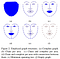A figure from (Antonakos et al., CVPR, 2015) showing representation of a face as a graph of landmarks. This is an interesting approach, but it is not a sufficient facial representation in many cases, since a lot can be told from the face texture captured well by convolutional networks. In contrast, reasoning over 3D meshes of a face looks like a more sensible approach compared to 2D landmarks (Ranjan et al., ECCV, 2018).

2. Why is it difficult to define convolution on graphs?

To answer this question, I first give some motivation for using convolution in general and then describe “convolution on images” using the graph terminology which should make the transition to “convolution on graphs” more smooth.

2.1. Why is convolution useful?

Let’s understand why we care about convolution so much and why we want to use it for graphs. Compared to fully-connected neural networks (a.k.a. NNs or MLPs), convolutional networks (a.k.a. CNNs or ConvNets) have certain advantages explained below based on the image of a nice old Chevy.

First, ConvNets exploit a natural prior in images, more formally described in (Bronstein et al., 2016), such as:

1. Shift-invariance — if we translate the car on the image above to the left/right/up/down, we still should be able to detect and recognize it as a car. This is exploited by sharing filters across all locations, i.e. applying convolution.
2. Locality — nearby pixels are closely related and often represent some semantic concept, such as a wheel or a window. This is exploited by using relatively large filters, which can capture image features in a local spatial neighborhood.
3. Compositionality (or hierarchy)— a larger region in the image is often a semantic parent of smaller regions it contains. For example, a car is a parent of doors, windows, wheels, driver, etc. And a driver is a parent of head, arms, etc. This is implicitly exploited by stacking convolutional layers and applying pooling.

Second, the number of trainable parameters (i.e. filters) in convolutional layers does not depend on the input dimensionality, so technically we can train exactly the same model on 28×28 and 512×512 images. In other words, the model is parametric.

Ideally, our goal is to develop a model that is as flexible as Graph Neural Nets and can digest and learn from any data, but at the same time we want to control (regularize) factors of this flexibility by turning on/off certain priors.

All these nice properties make ConvNets less prone to overfitting (high accuracy on the training set and low accuracy on the validation/test set), more accurate in different visual tasks, and easily scalable to large images and datasets. So, when we want to solve important tasks where input data are graph-structured, it is appealing to transfer all these properties to graph neural networks (GNNs) to regularize their flexibility and make them scalable. Ideally, our goal is to develop a model that is as flexible as GNNs and can digest and learn from any data, but at the same time we want to control (regularize) factors of this flexibility by turning on/off certain priors. This can open research in many interesting directions. However, controlling of this trade-off is challenging.

2.2. Convolution on images in terms of graphs

Let’s consider an undirected graph G with N nodes. Edges E represent undirected connections between nodes. Nodes and edges typically come from your intuition about the problem. Our intuition in the case of images is that nodes are pixels or superpixels (a group of pixels of weird shape) and edges are spatial distances between them. For example, the MNIST image below on the left is typically represented as an 28×28 dimensional matrix. We can also represent it as a set of N=28*28=784 pixels. So, our graph G is going to have N=784 nodes and edges will have large values (thicker edges in the Figure below) for closely located pixels and small values (thinner edges) for remote pixels.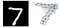An image from the MNIST dataset on the left and an example of its graph representation on the right. Darker and larger nodes on the right correspond to higher pixel intensities. The figure on the right is inspired by Figure 5 in (Fey et al., CVPR, 2018)

When we train our neural networks or ConvNets on images, we implicitly define images on a graph — a regular two-dimensional grid as the one on the figure below. Since this grid is the same for all training and test images and is regular, i.e. all pixels of the grid are connected to each other in exactly the same way across all images (i.e. have the same number of neighbors, length of edges, etc.), this regular grid graph has no information that will help us to tell one image from another. Below I visualize some 2D and 3D regular grids, where the order of nodes is color-coded. By the way, I’m using NetworkX in Python to do that, e.g. G = networkx.grid_graph([4, 4]).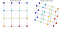Examples of regular 2D and 3D grids. Images are defined on 2D grids and videos are on 3D grids.

Given this 4×4 regular grid, let’s briefly look at how 2D convolution works to understand why it’s difficult to transfer this operator to graphs. A filter on a regular grid has the same order of nodes, but modern convolutional nets typically have small filters, such as 3×3 in the example below. This filter has 9 values: W₁,W₂,…, W₉, which is what we are updating during training using backprop to minimize the loss and solve the downstream task. In our example below, we just heuristically initialize this filter to be an edge detector (see other possible filters here):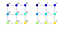Example of a 3×3 filter on a regular 2D grid with arbitrary weights w on the left and an edge detector on the right.

When we perform convolution, we slide this filter in both directions: to the right and to the bottom, but nothing prevents us from starting in the bottom corner — the important thing is to slide over all possible locations. At each location, we compute the dot product between the values on the grid (let’s denote them as X) and the values of filters, W: XW₁+XW₂+…+XW₉, and store the result in the output image. In our visualization, we change the color of nodes during sliding to match the colors of nodes in the grid. In a regular grid, we always can match a node of the filter with a node of the grid. Unfortunately, this is not true for graphs as I’ll explain later below.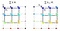2 steps of 2D convolution on a regular grid. If we don’t apply padding, there will be 4 steps in total, so the result will be a 2×2 image. To make the resulting image larger, we need to apply padding. See a comprehensive guide to convolution in deep learning here.

The dot product used above is one of so called “aggregator operators”. Broadly speaking, the goal of an aggregator operator is to summarize data to a reduced form. In our example above, the dot product summarizes a 3×3 matrix to a single value. Another example is pooling in ConvNets. Keep in mind, that such methods as max or sum pooling are permutation-invariant, i.e. they will pool the same value from a spatial region even if you randomly shuffle all pixels inside that region. To make it clear, the dot product is not permutation-invariant simply because in general: XW₁+XW₂ ≠XW₁+XW₂.

Now let’s use our MNIST image and illustrate the meaning of a regular grid, a filter and convolution. Keeping in mind our graph terminology, this regular 28×28 grid will be our graph G, so that every cell in this grid is a node, and node features are an actual image X, i.e. every node will have just a single feature — pixel intensity from 0 (black) to 1 (white).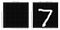Regular 28×28 grid (left) and an image on that grid (right).

Next, we define a filter and let it be a famous Gabor filter with some (almost) arbitrary parameters. Once we have an image and a filter, we can perform convolution by sliding the filter over that image (of digit 7 in our case) and putting the result of the dot product to the output matrix after each step.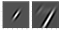A 28×28 filter (left) and the result of 2D convolution of this filter with the image of digit 7 (right).

This is all cool, but as I mentioned before, it becomes tricky when you try to generalize convolution to graphs.

Nodes are a set, and any permutation of this set does not change it. Therefore, the aggregator operator that people apply should be permutation-invariant.

As I have already mentioned, the dot product used above to compute convolution at each step is sensitive to the order. This sensitivity permits us to learn edge detectors similar to Gabor filters important to capture image features. The problem is that in graphs there is no well-defined order of nodes unless you learn to order them, or come up with some heuristic that will result in a consistent (canonical) order from graph to graph. In short, nodes are a set, and any permutation of this set does not change it. Therefore, the aggregator operator that people apply should be permutation-invariant. The most popular choices are averaging (GCN, Kipf & Welling, ICLR, 2017) and summation (GIN, Xu et al., ICLR, 2019) of all neighbors, i.e. sum or mean pooling, followed by projection by a trainable vector W. See Hamilton et al., NIPS, 2017 for some other aggregators.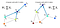Illustration of “convolution on graphs” of node features X with filter W centered at node 1 (dark blue).

For example, for the graph above on the left, the output of the summation aggregator for node 1 will be X₁=(X₁+X₂+X₃+X₄)W₁, for node 2: X₂=(X₁+X₂+X₃+X₅)W₁ and so forth for nodes 3, 4 and 5, i.e. we need to apply this aggregator for all nodes. In result, we will have the graph with the same structure, but node features will now contain features of neighbors. We can process the graph on the right using the same idea.

Colloquially, people call this averaging or summation “convolution”, since we also “slide” from one node to another and apply an aggregator operator in each step. However, it’s important to keep in mind that this is a very specific form of convolution, where filters don’t have a sense of orientation. Below I’ll show how those filters look like and give an idea how to make them better.

3. What makes a neural network a graph neural network?

You know how a classical neural network works, right? We have some C-dimensional features X as the input to the net. Using our running MNIST example, X will be our C=784 dimensional pixel features (i.e. a “flattened” image). These features get multiplied by C×F dimensional weights W that we update during training to get the output closer to what we expect. The result can be directly used to solve the task (e.g. in case of regression) or can be further fed to some nonlinearity (activation), like ReLU, or other differentiable (or more precisely, sub-differentiable) functions to form a multi-layer network. In general, the output of some layer l is: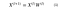Fully-connected layer with learnable weights W. “Fully-connected” means that each output value in X⁽ˡ⁺¹⁾ depends on, or “connected to”, all inputs X⁽ˡ⁾. Typically, although not always, we add a bias term to the output.

The signal in MNIST is so strong, that you can get an accuracy of 91% by just using the formula above and the Cross Entropy loss without any nonlinearities and other tricks (I used a slightly modified PyTorch example to do that). Such model is called multinomial (or multiclass, since we have 10 classes of digits) logistic regression.

Now, how do we transform our vanilla neural network to a graph neural network? As you already know, the core idea behind GNNs is aggregation over “neighbors”. Here, it is important to understand that in many cases, it is actually you who specifies “neighbors”.

Let’s consider a simple case first, when you are given some graph. For example, this can be a fragment (subgraph) of a social network with 5 persons and an edge between a pair of nodes denotes if two people are friends (or at least one of them think so). An adjacency matrix (usually denoted as A) in the figure below on the right is a way to represent these edges in a matrix form, convenient for our deep learning frameworks. Yellow cells in the matrix represent the edge and blue — the absence of the edge.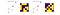Example of a graph and its adjacency matrix. The order of nodes we defined in both cases is random, while the graph is still the same.

Now, let’s create an adjacency matrix A for our MNIST example based on coordinates of pixels (complete code is provided in the end of the post):

import numpy as np
from scipy.spatial.distance import cdist
img_size = 28 # MNIST image width and height
col, row = np.meshgrid(np.arange(img_size), np.arange(img_size))
coord = np.stack((col, row), axis=2).reshape(-1, 2) / img_size
dist = cdist(coord, coord) # see figure below on the left
sigma = 0.2 * np.pi # width of a Gaussian
A = np.exp(- dist / sigma ** 2) # see figure below in the middle

This is a typical, but not the only, way to define an adjacency matrix for visual tasks (Defferrard et al., NIPS, 2016, Bronstein et al., 2016). This adjacency matrix is our prior, or our inductive bias, we impose on the model based on our intuition that nearby pixels should be connected and remote pixels shouldn’t or should have very thin edge (edge of a small value). This is motivated by observations that in natural images nearby pixels often correspond to the same object or objects that interact frequently (the locality principle we mentioned in Section 2.1.), so it makes a lot of sense to connect such pixels.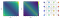Adjacency matrix (NxN) in the form of distances (left) and closeness (middle) between all pairs of nodes. (right) A subgraph with 16 neighboring pixels corresponding to the adjacency matrix in the middle. Since it’s a complete subgraph, it’s also called a “clique”.

So, now instead of having just features X we have some fancy matrix A with values in the range [0,1]. It’s important to note that once we know that our input is a graph, we assume that there is no canonical order of nodes that will be consistent across all other graphs in the dataset. In terms of images, it means that pixels are assumed to be randomly shuffled. Finding the canonical order of nodes is combinatorially unsolvable in practice. Even though for MNIST we technically can cheat by knowing this order (because data are originally from a regular grid), it’s not going to work on actual graph datasets.

Remember that our matrix of features X has 𝑁 rows and C columns. So, in terms of graphs, each row corresponds to one node and C is the dimensionality of node features. But now the problem is that we don’t know the order of nodes, so we don’t know in which row to put features of a particular node. If we just pretend to ignore this problem and feed X directly to an MLP as we did before, the effect will be the same as feeding images with randomly shuffled pixels with independent (yet the same for each epoch) shuffling for each image! Surprisingly, a neural network can in principle still fit such random data (Zhang et al., ICLR, 2017), however test performance will be close to random prediction. One of the solutions is to simply use the adjacency matrix A, we created before, in the following way: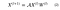Graph neural layer with adjacency matrix A, input/output features X and learnable weights W.

We just need to make sure that row i in A corresponds to features of node in row i of X. Here, I’m using 𝓐 instead of plain A, because often you want to normalize A. If 𝓐=A, the matrix multiplication 𝓐X⁽ˡ⁾ will be equivalent to summing features of neighbors, which turned out to be useful in many tasks (Xu et al., ICLR, 2019). Most commonly, you normalize it so that 𝓐X⁽ˡ⁾ averages features of neighbors, i.e. 𝓐=A/ΣᵢAᵢ. A better way to normalize matrix A can be found in (Kipf & Welling, ICLR, 2017).

Below is the comparison of NN and GNN in terms of PyTorch code:

And here is the full PyTorch code to train two models above: python mnist_fc.py --model fc to train the NN case; python mnist_fc.py --model graph to train the GNN case. As an exercise, try to randomly shuffle pixels in code in the --model graph case (don’t forget to shuffle A in the same way) and make sure that it will not affect the result. Is it going to be true for the --model fc case?

After running the code, you may notice that the classification accuracy is actually about the same. What’s the problem? Aren’t graph networks supposed to work better? Well, they are, in many cases. But not in this one, because the 𝓐X⁽ˡ⁾ operator we added is actually nothing else, but a Gaussian filter: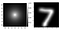2D visualization of a filter used in a graph neural network and it’s effect on the image.

So, our graph neural network turned out to be equivalent to a convolutional neural network with a single Gaussian filter, that we never update during training, followed by the fully-connected layer. This filter basically blurs/smooths the image, which is not a particularly useful thing to do (see the image above on the right). However, this is the simplest variant of a graph neural network, which nevertheless works great on graph-structured data. To make GNNs work better on regular graphs, like images, we need to apply a bunch of tricks. For example, instead of using a predefined Gaussian filter, we can learn to predict an edge between any pair of pixels by using a differentiable function like this:

import torch.nn as nn  # using PyTorchnn.Sequential(nn.Linear(4, 64),  # map coordinates to a hidden layer
nn.ReLU(), # nonlinearity
nn.Linear(64, 1), # map hidden representation to edge
nn.Tanh()) # squash edge values to [-1, 1]

To make GNNs work better on regular graphs, like images, we need to apply a bunch of tricks. For example, instead of using a predefined Gaussian filter, we can learn to predict an edge between any pair of pixels.

This idea is similar to Dynamic Filter Networks (Brabander et al., NIPS, 2016), Edge-conditioned Graph Networks (ECC, Simonovsky & Komodakis, CVPR, 2017) and (Knyazev et al., NeurIPS-W, 2018). To try it using my code, you just need to add the --pred_edge flag, so the entire command is python mnist_fc.py --model graph --pred_edge. Below I show the animation of the predefined Gaussian and learned filters. You may notice that the filter we just learned (in the middle) looks weird. That’s because the task is quite complicated since we optimize two models at the same time: the model that predicts edges and the model that predicts a digit class. To learn better filters (like the one on the right), we need to apply some other tricks from our BMVC paper, which is beyond the scope of this part of the tutorial.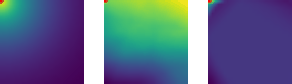2D filter of a graph neural network centered in the red point. Averaging (left, accuracy 92.24%), learned based on coordinates (middle, accuracy 91.05%), learned based on coordinates with some tricks (right, accuracy 92.39%).

The code to generate these GIFs is quite simple:

I’m also sharing an IPython notebook showing 2D convolution of an image with a Gabor filter in terms of graphs (using an adjacency matrix) compared to using circulant matrices, which is often used in signal processing.

In the next part of the tutorial, I’ll tell you about more advanced graph layers that can lead to better filters on graphs.

Conclusion

Graph Neural Networks are a very flexible and interesting family of neural networks that can be applied to really complex data. As always, such flexibility must come at a certain cost. In case of GNNs it is the difficulty of regularizing the model by defining such operators as convolution. Research in that direction is advancing quite fast, so that GNNs will see application in increasingly wider areas of machine learning and computer vision.

Acknowledgement: A large portion of this tutorial was prepared during my internship at SRI International under the supervision of Mohamed Amer (homepage) and my PhD advisor Graham Taylor (homepage).

Find me on Github, LinkedIn and Twitter.

Written by

Boris Knyazev

PhD student at University of Guelph

Welcome to a place where words matter. On Medium, smart voices and original ideas take center stage - with no ads in sight. Watch
Follow all the topics you care about, and we’ll deliver the best stories for you to your homepage and inbox. Explore
Get unlimited access to the best stories on Medium — and support writers while you’re at it. Just \$5/month. Upgrade